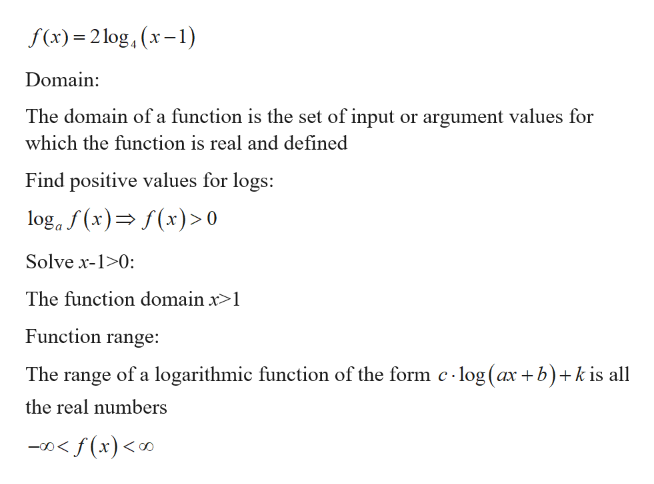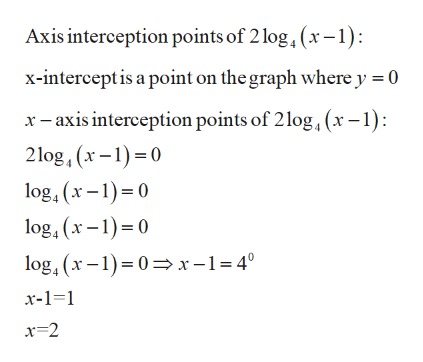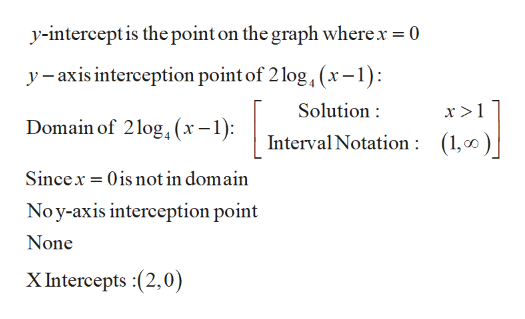Question
1 views

how do i graph the log function below , and include the vertical asymptote and key points on the graph ? step by step please.

check_circle

Step 1

Given function:help_outlineImage Transcriptionclosef(x) = 2 log, (x– 1) Domain: The domain of a function is the set of input or argument values for which the function is real and defined Find positive values for logs: log, f (x)= f(x)> 0 Solve x-1>0: The function domain x>1 Function range: The range of a logarithmic function of the form c - log (ax +b)+k is all the real numbers -0< f(x)<∞ fullscreen
Step 2

Step 1 as followshelp_outlineImage TranscriptioncloseAxis interception points of 2 log, (x-1): x-intercept is a point on the graph where y = 0 x- axis interception points of 2log, (x – 1): 2log, (x-1)=0 log, (x – 1)= 0 log, (x – 1)= 0 log, (x-1)=0=x-1=4° x-1=1 x=2 fullscreen
Step 3

Step 2 as fo...help_outlineImage Transcriptionclosey-intercept is the point on the graph where.x = 0 y - axis interception point of 2 log,(x-1): Solution : x >1 Domain of 2log,(x-. –1): Interval Notation : (1,0)| Sincex = 0is not in domain No y-axis interception point None X Intercepts :(2,0) fullscreen

### Want to see the full answer?

See Solution

#### Want to see this answer and more?

Solutions are written by subject experts who are available 24/7. Questions are typically answered within 1 hour.*

See Solution
*Response times may vary by subject and question.
Tagged in

### Calculus